Data Interpretation MCQ -5

# Data Interpretation MCQ -5

Test Description

## 20 Questions MCQ Test Quantitative Techniques for CLAT | Data Interpretation MCQ -5

Data Interpretation MCQ -5 for Railways 2023 is part of Quantitative Techniques for CLAT preparation. The Data Interpretation MCQ -5 questions and answers have been prepared according to the Railways exam syllabus.The Data Interpretation MCQ -5 MCQs are made for Railways 2023 Exam. Find important definitions, questions, notes, meanings, examples, exercises, MCQs and online tests for Data Interpretation MCQ -5 below.
Solutions of Data Interpretation MCQ -5 questions in English are available as part of our Quantitative Techniques for CLAT for Railways & Data Interpretation MCQ -5 solutions in Hindi for Quantitative Techniques for CLAT course. Download more important topics, notes, lectures and mock test series for Railways Exam by signing up for free. Attempt Data Interpretation MCQ -5 | 20 questions in 15 minutes | Mock test for Railways preparation | Free important questions MCQ to study Quantitative Techniques for CLAT for Railways Exam | Download free PDF with solutions
 1 Crore+ students have signed up on EduRev. Have you?
Data Interpretation MCQ -5 - Question 1

### (Q.no: 1-5). Refer to the following Line Graph and answer the given questions.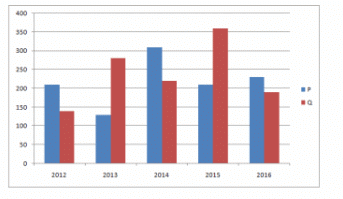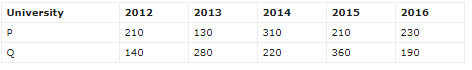In 2013, out of the total number of applications received for Universities P and Q together, only 30% were accepted. What was the total number of applications accepted for Universities P and Q together in 2013?

Detailed Solution for Data Interpretation MCQ -5 - Question 1

Total number of applications accepted by P and Q together in 2013 = (130 + 280)*30/100
=123

Data Interpretation MCQ -5 - Question 2

### (Q.no: 1-5). Refer to the following Line Graph and answer the given questions.In 2012, 30% of applications received for University P and 20% of applications received for University Q were from international students. What was the total number of International applicants for University P and Q together in 2012?

Detailed Solution for Data Interpretation MCQ -5 - Question 2

Total Number of International applicants for University P and Q together = 210*30/100 + 140 * 20/100
= 63 + 28 = 91

Data Interpretation MCQ -5 - Question 3

### (Q.no: 1-5). Refer to the following Line Graph and answer the given questions.If the respective ratio of total number of applications received for University P and Q together in 2016 and 2017 is 3:4. What was the total number of applications received for University P and Q together in 2017?

Detailed Solution for Data Interpretation MCQ -5 - Question 3

Total number of applications received for Universities P and Q together in 2016 = 230 + 190 = 420
Total number of applications received for Universities P and Q together in 2017 = 420 * 4/3 = 560

Data Interpretation MCQ -5 - Question 4

(Q.no: 1-5). Refer to the following Line Graph and answer the given questions.What is the average number of applications received for University P in 2013, 2015 and 2016?

Detailed Solution for Data Interpretation MCQ -5 - Question 4

Average number of applications received for University A in 2013, 2015 and 2016 = (130 + 210 + 230)/3 = 190

Data Interpretation MCQ -5 - Question 5

(Q.no: 1-5). Refer to the following Line Graph and answer the given questions.Number of applications received for University Q increased by what percent from 2012 to 2014?

Detailed Solution for Data Interpretation MCQ -5 - Question 5

% = (220-140)/140 * 100 = 400/7 %

Data Interpretation MCQ -5 - Question 6

(Q.no: 6-10). Refer to the following Line Graph and answer the given questions.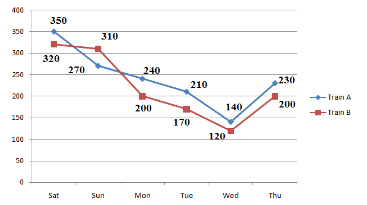The number of people who traveled by Train B on Friday is 30% more than the people who traveled by the same train on Thursday. What is the respective ratio between the number of people who traveled on Friday and those who traveled on Saturday by the same train?

Detailed Solution for Data Interpretation MCQ -5 - Question 6

30% of 200 = 60
People traveled by Train B on Friday = 260
260 : 320 = 13 : 16

Data Interpretation MCQ -5 - Question 7

(Q.no: 6-10). Refer to the following Line Graph and answer the given questions.What is the difference between the total number of people who traveled by Train B on Saturday and Sunday together and the total number of people who traveled by Train A on Saturday and Sunday together?

Detailed Solution for Data Interpretation MCQ -5 - Question 7

Train B on Saturday and Sunday together = 320 + 310 = 630
Train A on Saturday and Sunday together = 350 + 270 = 620
Difference = 630 – 620 = 10

Data Interpretation MCQ -5 - Question 8

(Q.no: 6-10). Refer to the following Line Graph and answer the given questions.What is the average number of people traveling by Train B on Monday, Tuesday, Wednesday and Thursday?

Detailed Solution for Data Interpretation MCQ -5 - Question 8

200 + 170 + 120 + 200
= 172.5

Data Interpretation MCQ -5 - Question 9

(Q.no: 6-10). Refer to the following Line Graph and answer the given questions.The number of people who traveled by Train A decreased by what percent from Saturday to Wednesday?

Detailed Solution for Data Interpretation MCQ -5 - Question 9

[(350 – 140)/350]*100
= [210/350]*100 = 60%

Data Interpretation MCQ -5 - Question 10

(Q.no: 6-10). Refer to the following Line Graph and answer the given questions.The total number of people who traveled by both the given trains together on Monday is approximately what percent more than the total number of people who traveled by both the given trains together on Wednesday?

Detailed Solution for Data Interpretation MCQ -5 - Question 10

(440 – 260)/260*100
= 180/260 * 100
= 69%

Data Interpretation MCQ -5 - Question 11

(Q.no: 15-20). Refer to the table and answer the given questions.
Total Population in Five Cities = 15,00,000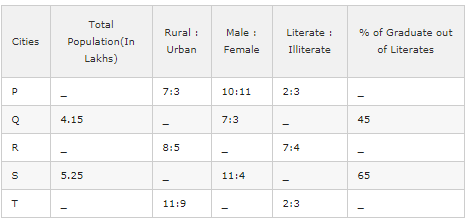The total population of City P and R is 7,85,000. The difference between the total population of City P and R is 2.65 lakhs. If the City P has more population than City R then the urban population of City P and R together is approximately what percent of total population of these two Cities?

Detailed Solution for Data Interpretation MCQ -5 - Question 11

P + R = 7,85,000
(R has more population than P) R – P = 265000
P = 5,25,000 ; R = 2,60,000
Urban population of City P and R = 3/10 * 5,25,000 + 5/13 * 2,60,000 = 257500
% = (257500 / 785000)*100 = 32%

Data Interpretation MCQ -5 - Question 12

(Q.no: 15-20). Refer to the table and answer the given questions.
Total Population in Five Cities = 15,00,000The difference between the number of male and female in the City P is 10000. Approximately, by what percent of urban population of City P less than its Rural population?

Detailed Solution for Data Interpretation MCQ -5 - Question 12

X = Total population in City A
1/21 * x = 10000 => x = 2,10,000
Rural Population = 1,47,000
Urban Population = 63,000
% = (Difference / Rural Population)*100 = (84,000 / 147000)*100 = 57%

Data Interpretation MCQ -5 - Question 13

(Q.no: 15-20). Refer to the table and answer the given questions.
Total Population in Five Cities = 15,00,000One – fourth of total population of City R is 2,20,000.  If the total number of Literate graduates of City Q and S are 22,500 and 52,000. What % of the total population of City Q, R and S together is literate?

Detailed Solution for Data Interpretation MCQ -5 - Question 13

1/4 * R = 2,20,000 => R = 8,80,000
Literate population of City R = 7/11 * 8,80,000 = 5,60,000
Total number of Literate graduates of City Q = 22500 = 45%
x = Literate population of City Q
45% of x = 22500 => x = 50000
Total number of Literate graduates of City S = 52000 = 65%
y = Literate population of City S
65% of y = 52000 => x = 80000
% = (690000/1820000) * 100 = 37.9%

Data Interpretation MCQ -5 - Question 14

(Q.no: 15-20). Refer to the table and answer the given questions.
Total Population in Five Cities = 15,00,000The difference between the total population of City R and T is 30,000. The ratio of total population of City R and T is 44:41. Total % of Literate graduates of City R and T is 90%. If the % Literate graduates of City T is equalled to two times of Literate graduates of City R then what is the difference between the number of graduates from City R and City T?

Detailed Solution for Data Interpretation MCQ -5 - Question 14

3/85 * x = 30000 => x = 850000
44/85*850000 = 440000 = City R Population
7/11*440000 = 280000
Number of graduates from City R = 30% of 280000 = 84000
41/85*85000 = 410000 = City T Population
2/5*410000 = 164000
Number of graduates from City T = 60% of 1,64,000 = 98400
Difference = 14400

Data Interpretation MCQ -5 - Question 15

(Q.no: 15-20). Refer to the table and answer the given questions.
Total Population in Five Cities = 15,00,000What is the sum of female population in City Q and male population in City S together ?

Detailed Solution for Data Interpretation MCQ -5 - Question 15

Sum = 3/10 * 415000 + 11/15 *525000 = 124500 + 385000 = 509500

Data Interpretation MCQ -5 - Question 16

(Q.no: 16 -20). Refer to the table and answer the given questions.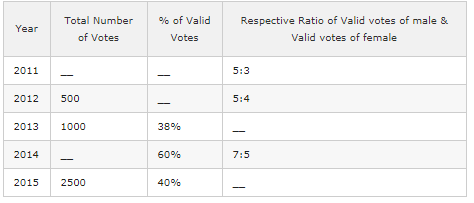In 2013, if the number of valid votes of female was 100, what was the respective ratio of number of valid votes of male and number of valid votes of female same year?

Detailed Solution for Data Interpretation MCQ -5 - Question 16

38% of 1000 = 380
Valid votes of Female = 100
Valid votes of Male = 380 – 100 = 280
=> 280:100
=> 14:5

Data Interpretation MCQ -5 - Question 17

(Q.no: 16 -20). Refer to the table and answer the given questions.The total number of votes increased by 50% from 2012 to 2016. If 16% of the votes valid in 2016, what was the number of valid votes in 2016?

Detailed Solution for Data Interpretation MCQ -5 - Question 17

50% of 500 = 250
= 500 + 250 = 750
16% of 750 = 120

Data Interpretation MCQ -5 - Question 18

(Q.no: 16 -20). Refer to the table and answer the given questions.If the average number of valid votes in 2012 and 2015 was 635, approximately, what percent of total votes valid in 2012?

Detailed Solution for Data Interpretation MCQ -5 - Question 18

Number of valid votes in 2012 and 2015 = 1270
2015: 40% of 2500 = 1000
1270 – 1000 = 270
(270/500)*100 = 54%

Data Interpretation MCQ -5 - Question 19

(Q.no: 16 -20). Refer to the table and answer the given questions.In 2014, if the difference between number of valid votes of male and number of valid votes of female was 150, what was the total number of votes in 2014?

Detailed Solution for Data Interpretation MCQ -5 - Question 19

(1/6)*x = 15
x = 900
60% of y = 900
y = 1500

Data Interpretation MCQ -5 - Question 20

(Q.no: 16 -20). Refer to the table and answer the given questions.In 2011, the respective ratio of total number of votes to valid votes was 5:4. Number of valid votes of female in 2011 constitutes what percent of the total number of votes in the same year?

Detailed Solution for Data Interpretation MCQ -5 - Question 20

[4x * (3/8) / 5] * 100 = 30%

## Quantitative Techniques for CLAT

56 videos|35 docs|91 tests
 Use Code STAYHOME200 and get INR 200 additional OFF Use Coupon Code
Information about Data Interpretation MCQ -5 Page
In this test you can find the Exam questions for Data Interpretation MCQ -5 solved & explained in the simplest way possible. Besides giving Questions and answers for Data Interpretation MCQ -5, EduRev gives you an ample number of Online tests for practice

## Quantitative Techniques for CLAT

56 videos|35 docs|91 tests